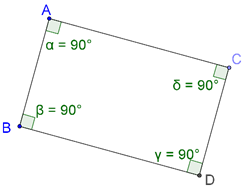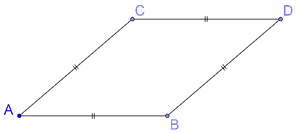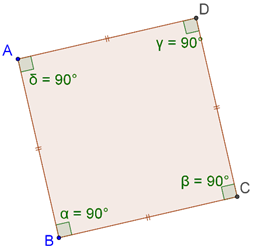# Parallelograms

Go back to  'Triangles-Quadrilaterals'

We will now consider some particular kinds of parallelograms.

## Rectangle

A rectangle is a parallelogram in which adjacent sides are perpendicular to each other. In the following figure, ABCD is a rectangle:Recall that the area of a parallelogram is the product of its length and breadth.

## Rhombus

A rhombus is a parallelogram in which all the four sides are equal, as shown below:## Square

A square is a rectangle which is also a rhombus. In other words, in a square, adjacent sides are perpendicular to each other, and all the four sides are equal: